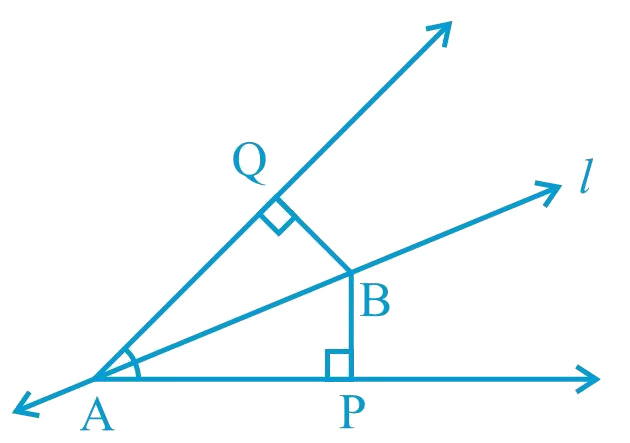# Ex.7.1 Q5 Triangles Solution - NCERT Maths Class 9

Go back to  'Ex.7.1'

## Question

Line $$l$$ is the bisector of an angle $$\angle A$$ and $$\angle B$$ is any point on $$l$$. $$BP$$ and $$BQ$$ are perpendiculars from $$B$$ to the arms of $$\angle A$$ (see the given figure).

Show that:

(i) $$\Delta APB \cong \Delta AQB$$

(ii) $$BP = BQ$$ or $$B$$ is equidistant from the arms of $$\angle A$$.

Video Solution
Triangles
Ex 7.1 | Question 5

## Text Solution

What is known?

$$l$$ is the bisector of an angle $$\angle {\text{A}}$$ and $$BP \bot AP\;{\text{and BQ}} \bot {\text{AQ}}$$

To prove:

$$\Delta {\text{APB}} \cong \Delta {\text{AQB}}$$ and $${\text{BP}}\,{\text{ = }}\,{\text{BQ}}$$ or B is equidistant from the arms of $$\angle {\text{A}}$$

Reasoning:

We can show two triangles APB and AQB congruent by using AAS congruency rule and then we can say corresponding parts of congruent triangles will be equal.Steps:

In $$\Delta APB$$ and $$\Delta AQB$$,

\begin{align}&\angle BAP= \angle BAQ \\&\text{(l) is the angle bisector of }(\angle A)\\\\&\angle APB = AQB ( \text{Each} \;90^ {\circ})\\AB&=AB \text{(Common)}\\&\therefore \Delta APB \cong \Delta AQB \\&\text{(By AAS congruence rule)}\\\\\therefore BP &= BQ (By CPCT)\end{align}

Or, it can be said that $$B$$ is equidistant from the arms of $$\angle A$$.

Learn from the best math teachers and top your exams

• Live one on one classroom and doubt clearing
• Practice worksheets in and after class for conceptual clarity
• Personalized curriculum to keep up with school